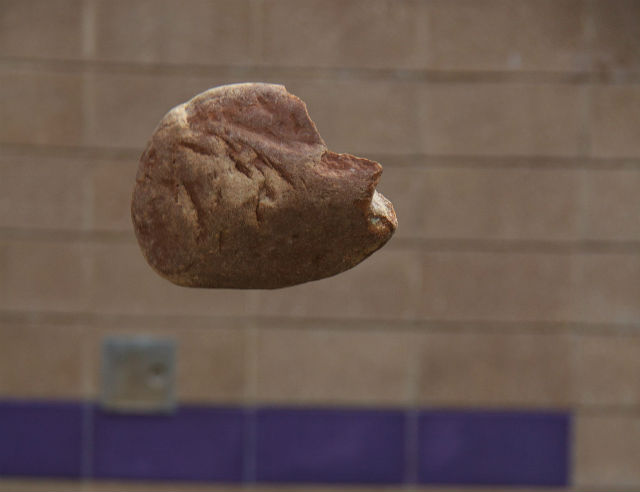# Solution to problems – class 9 – Set 1 Q37, 38, 39, 40, 41

Last updated on June 17th, 2019 at 02:47 pm

solution of 5 numericals from Q set 1 for class 9. Motion under gravity is the primary focus of the following problems.

Upward and downward movement, maximum height reached, time to reach the maximum height, velocity just before touching the ground etc are the touched upon points of these sort of numerical problems in physics. You can get the question set here

## icse problems for class 9 physics – physics question

37) A piece of stone is thrown vertically upwards. It reaches the maximum height in 3 seconds. If the acceleration of the stone be 9.8 m/s^2 directed towards the ground, calculate the initial velocity of the stone with which it is thrown upwards.

Solution
Time to reach the max height = T=3 sec

let the initial upward velocity = u

As per formula, T = u/g

That means, u/g = 3

So, u = 3 g = 3 x 9.8 = 29.4 m/s

38) A stone falls from a building and reaches the ground 2.5 seconds later. How high is the building ? (g = 9.8 m/s^2)

##### icse problems for class 9 physics – physics question

Solution
During downward movement from rest, the initial velocity is u = 0

So the equation becomes:
H = (1/2) g t2 = (1/2) x 9.8 x 2.52 = 30.6 m

39) A stone is dropped from a height of 20 m.(i) How long will it take to reach the ground?(ii) What will be its speed when it hits the ground? (g = 10 m/s^2)

##### icse problems for class 9 physics – physics question

Solution

H = 20 m
For downward movement from rest, U=0
To find out t, we will use the eqn, V = gt
so, t = V/g ….. (1)

As yet we don’t know the value of V, to find it out we have to use another eqn.
V2 = 2 g H
So, V2 = (2 x 9.8 x 20)
V = 19.8 m/s

So from eqn 1 we get t = V/g = 19.8/9.8 = 2 secs (approx) [ answer of i]
Final velocity as found above=19.8 m/s [answer of ii]

See also  Solution to problems - class 9 physics - Set 1 Q 53
##### physics problem solution – motion under gravity

40) A stone is thrown vertically upwards with a speed of 20 m/s. How high will it go before it begins to fall ? (g = 9.8 m/s^2)

Solution

The max height reached before it starts to fall = H = U2/(2 g) = 202/(2 x 9.8)= 20.4 m

##### icse problems for class 9 physics – physics question

41) When a cricket ball is thrown vertically upwards, it reaches a maximum height of 5 metres.(a) What was the initial speed of the ball ?(b) How much time is taken by the ball to reach the highest point ? (g =10 m/ s^2)

Solution

(a)At maximum height V =0
So from the eqn, V2 =U2 – 2gh

We get, U = (2 g h)1/2 = ( 2  x 10 x 5)1/2 = 10 m/s

(b)The time required to reach the max height = t = U/g = 10/10 =1 sec

Scroll to top
error: physicsTeacher.in